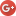## Aleksandr V. Bukalove-mail: boukalov@gmail.com
The Centre of Physical and Space Researches, International Institute of Socionics
"Physics of Consciousness and Life, Cosmology and Astrophysics" journal, issue 1, 2002 .

# Determination of the Hubble parameter exact value

The formula, obtained under the Universe quantum description, connects the values of the Universe mass and Hubble parameter H0 and allows to calculate them. Exact value of the Hubble's parameter was determined as H0=68.658±0.1 km·s-1·Mpc-1, that is in excellent agreement with the experimental data. Product G·MU changes proportionally to the Hubble time change: G·MU~t, which follows from the obtained formula. The discretion and limitation of energy of the Universe eliminates the Λ-problem, and calculated density of vacuum energy corresponds to the observed one.
Key words: quantum cosmology, vacuum of the Universe, harmonic oscillator, circular oscillation frequency, Hubble parameter.

It is well known that the exact value of the Hubble parameter is not theoretically calculated still. Meanwhile the Hubble parameter H0 is directly connected to the age of the Universe, and its determination is very important for the understanding of the Universe evolution.

The experimental determination of the Hubble parameter is connected to the objective difficulties, and permanent efforts of different research groups give the rather dispersed values. However, a method of the theoretical exact determination of the Hubble parameter could be proposed.

Now the Universe is described not only classically by the General Relativity, but also in the bounds of quantum cosmology, in the theories of the quantum universe birth from the vacuum, including inflationary cosmology [1, 2, 3].

According to A.Sacharov  the gravity is connected to the elasticity of vacuum of the elementary particles, therefore we can consider own the oscillations of the elastic vacuum of the Universe as an united whole and that oscillations (at least some of them) correspond to oscillations determined from the General Relativity.

Since gravitational radius of the closed Universe (Ω=1.02±0.02 ) accordingly to the gravitational theory of Einstein makes up
RG=2GMU /c2=8.46·1023m,   (1)
where MU=5.70·1053kg is the mass of the closed Universe , the according time of extension or compression makes
TG=RG /c=2.818·1018s,   (2)
and the pulsation period is Tp=2TG=5.636·1018s.

If we consider the elastic universe, filled up with a vacuum (possibly scalar) field, as a harmonic oscillator with the equation of movement,     (3)
then the oscillation period is determined from the condition of periodicity. The period T0 is known to determine the natural frequency ν0 and natural angular frequency ω0:(4)

For the quantum vacuum universe the frequencycorresponds to the zero vacuum oscillations, and the oscillation period is.     (5)

Thus, if the period of the natural Universe oscillations is accepted to be, then the natural angular frequency of Universe oscillations is(6)
for G= 6.673±0,01·10-11m3/s·kg .

Then(7)

Taking into account that by the latest datakm·s-1·Mpc-1 and according to the WMAP data, the estimation, obtained by method of maximum likelihood, is H0=68 km·s-1·Mpc-1 , and the counted frequency is ω0=68.658 km·s-1·Mpc-1, it is possible to identify the Hubble parameter value with the obtained natural angular frequency of the Universe oscillations:.    (8)

This means that the Hubble parameter can be calculated with high accuracy, which is considerably higher than experimental accuracy, but perfectly accords with it. In its turn, the formula (8) also allows to calculate the mass of the Universe:.    (9)

The mass, on the basis of experimental data on H0, makes upg, which corresponds to the Universe mass, determined in General Relativity,g , and to the obtained value.

However, Hubble parameter is a function of time. Then we obtain (with c=const) the dependence of G·MU product on time:
G·MU~t.     (10)
Results from (10) were discussed in particular article.

If vacuum energy density is set by vacuum field, then according to Zeldovich :With the obtained H0 value the critical density of the Universe makes.    (11)
Then for=42.25 MeV≈; when≈40 MeV=The given results show that the Universe energy could correspond to the discrete energy level of the macroscopic coherence field.

Then in the simplest case the Universe could be described by the Schrödinger equation for one-dimensional harmonic oscillator:(12)
with the energy levels, when the constantis a cosmological analogue of the Plank constantfor the Universe as a complete macroquantum object.

The given results show that the Universe could be considered as macroscopic quantum state of a coherence field, which is described by the equation for harmonic oscillator.

If the Universe energy corresponds to zero energy, then.

Then, probably, another energy levels of single Universe correspond to different macroscopic energy levels (n=1,2,3,…) of macroscopic coherent field, or to existence of another universes. If energy of universe corresponds to difference of adjacent energy levels of that field, then. In that case, process of universe genesis is equivalent to macroquantum particle emanation.

It is wonderful, that a simple model of quantum oscillator gives the exact results. This gives evidence about the quantum nature of Universe, filled up with a coherent vacuum (possibly scalar) field. Note, that the quantilization of universe energy, probably, automatically eliminates the known vacuum energy problem - the cosmological constant problem (Λ-problem).

Mass and energy of universeare limited, which limits the vacuum energy density and brings it down to observed value (11), that is 10122 times less then the quantum field theory predict .

Like an introduction of a quantum of action by M.Plank in 1900 allowed the explanation of the experimental data on electromagnetic radiation, the consideration of the Universe as a quantum object allows the calculation of the Hubble parameter and other cosmological parameters.

References:

1. Linde A.D. Particle physics and inflationary cosmology. (Boston,MA: Harvard University Press) (1990).
2. Fomin P.I. Preprint ITEP-73-1379. Kiev (1973).
3. Tryon E.P. Nature. 246, 396 (1973).
4. Sacharov A.D. RAS USSR, 177, 70 (1967).
5. Bukalov A.V. Proc. 2-nd Conf. "Gravitation, Cosmology and Relativistic Astrophysics" (Kharkov National University) 93 (2003).
6. CODATA http://www.codata.org
7. Spergel D.N. et al., astro-ph/0302209.
8. Misner C., Thorne K. and Wheeler J. Gravitation, (London: Freeman) (1973).
9. Zeldovich Y.B. JETP lett. 6, 345 (1967).
10. Weinberg S. Rev. Mod. Phys., 61, 1 (1989).